Related Articles

# How to Insert a Function in Excel?

• Last Updated : 14 Jul, 2021

MS Excel has many inbuilt in functions that we can use in our formula. If you want to see all the functions by category choose Formulas Tab and then Insert Function. Then Insert function Dialog appears from which we can choose function.

Some important functions of Excel by categories are:

Text Functions

• Lower: Converts all characters in a given text string to lowercase.
• UPPER: Converts all characters in a given text string to uppercase
• CONCATENATE: It will join together two or more text strings
• LEFT: It will return a specified number of characters from the beginning of a supplied text string
• RIGHT: Returns a specified number of characters from the end of a supplied text

Date & Time

• TIME: It will return a time, from a user-given hour, minute, and second.
• NOW: It will return the current date & time
• TODAY: It will return current today’s date.

Statistical

• SUM: It will return the sum of a given list of numbers
• MAX: It will return the largest value from a list of given numbers
• COUNTIF: It will return the number of cells (of a supplied range), that satisfy a given criterion.

Logical

• AND: Tests a number of user-defined conditions and returns TRUE if ALL of the conditions evaluate to TRUE, or FALSE otherwise
• OR: Tests a number of user-defined conditions and returns TRUE if ANY of the conditions evaluate to TRUE, or FALSE otherwise
• NOT: It will return a logical value that is the opposite of a user given logical value or expression i.e. returns FALSE is the given argument is TRUE and returns TRUE if the given argument is FALSE)

Mathematical

• SQRT: It will return the positive square root of a given number
• POWER: It will return the power of a number raised to the second number.

### How to Insert a function in Excel

Every function has the same structure. For example, SUM(A1:A4). The name of this function is SUM. The part between the brackets (arguments) means we’re giving Excel the range A1:A4 as input. This function adds the value in cells A1, A2, A3, and A4. It’s not easy to reminder which function and which arguments to use for each task. Fortunately, the Insert Function features in Excel help you with this.

To insert SUM function, need to follow the following steps:

Step 1. Select a cell.

Step 2. Click the Insert Function button. The ‘Insert Function‘ dialog box appears.

Step 3. Look for a function or select a function from a category. For example, choose SUM from the statistical category.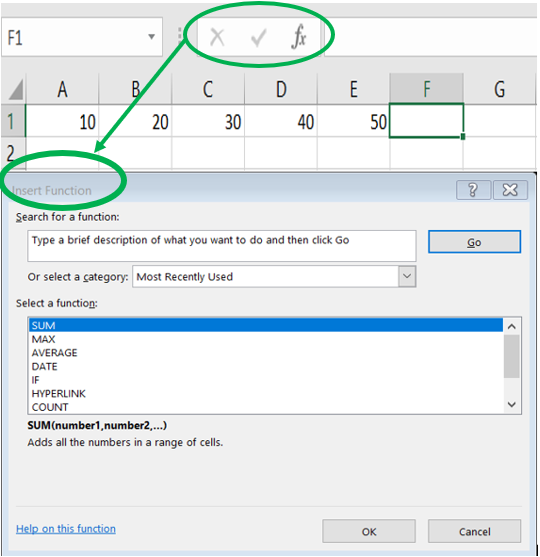Step 4. Click OK.

The ‘Function Arguments‘ dialog box appears.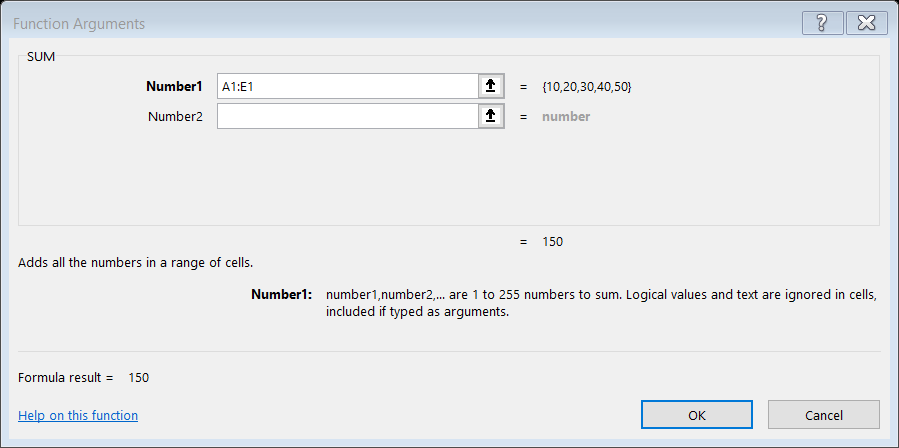Step 5. Click within the Range box and select the range A1:E1.

Step 6. Click OK.

Result: Excel will sum all the number which are present from A1:E1.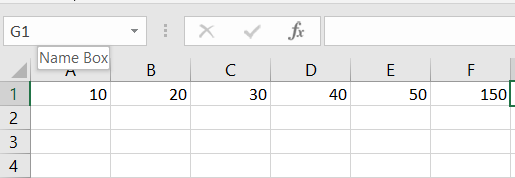To insert MAX function, need to follow the following steps:

Step 1. Select a cell.

Step 2. Click the Insert Function button. The ‘Insert Function‘ dialog box appears.

Step 3. Look for a function or select a function from a category. For example, choose MAX from the statistical category.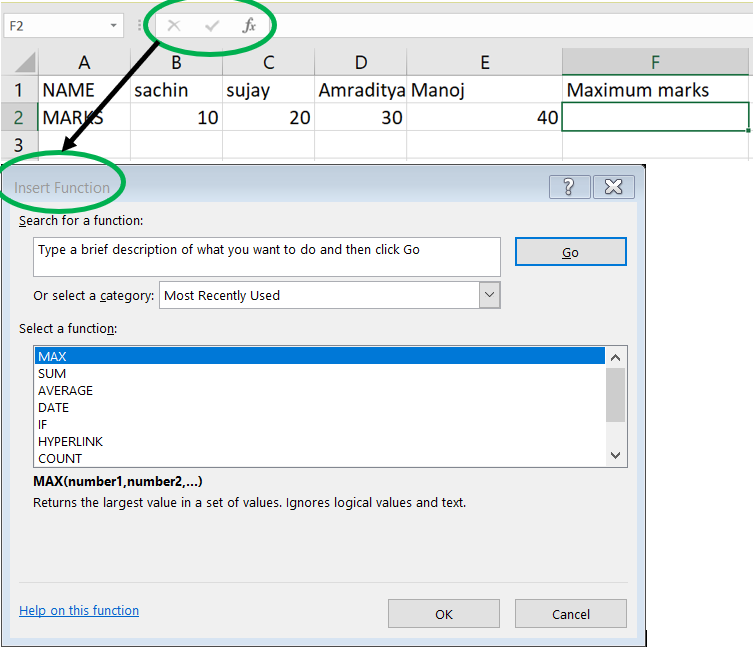Step 4. Click OK. The ‘Function Arguments‘ dialog box appears.

Step 5. Click within the Range box and select the range A1:E1.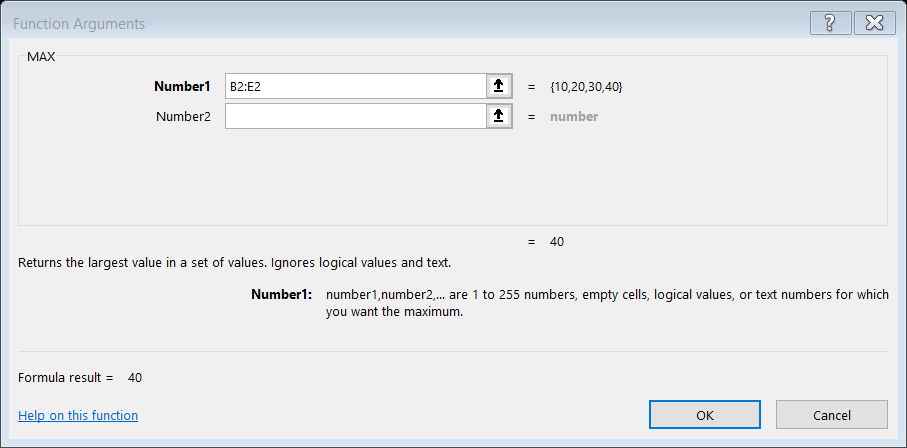Step 6. Click OK

Result: Excel will MAX all the number which are present from B2:E21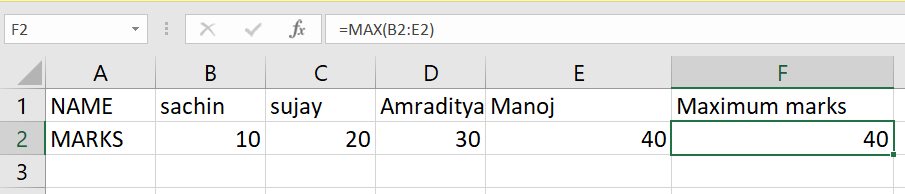To insert COUNT function, need to follow the following steps:

Step 1. Select a cell.

Step 2. Click the Insert Function button. The ‘Insert Function‘ dialog box appears.

Step 3. Look for a function or select a function from a category. For example, choose COUNT from the statistical category.Step 4. Click OK.

The ‘Function Arguments‘ dialog box appears.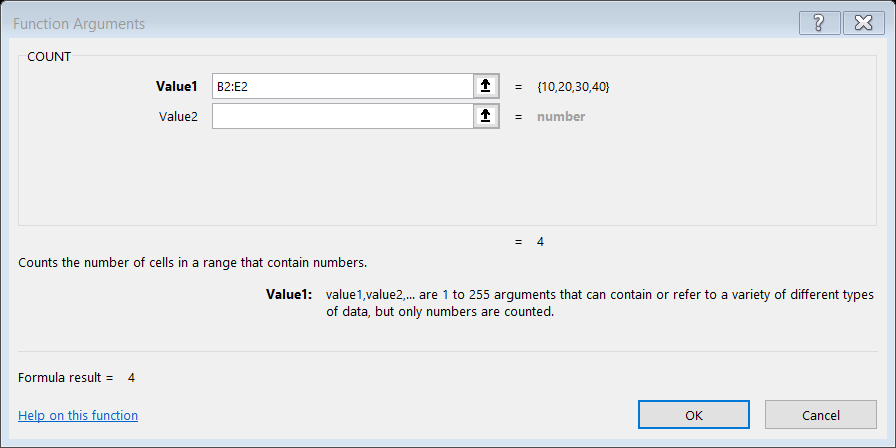Step 5. Click within the Range box and select the range B2:E2.

Step 6. Click OK.

Result: Excel will COUNT all the number which are present from B2:E2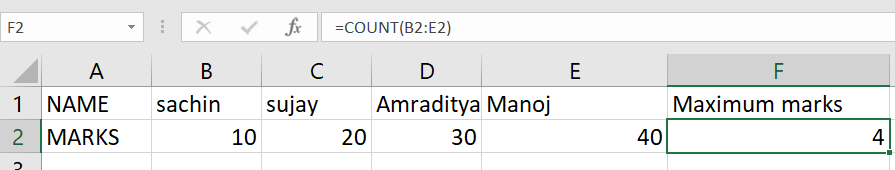Attention reader! Don’t stop learning now. Join the First-Step-to-DSA Course for Class 9 to 12 students , specifically designed to introduce data structures and algorithms to the class 9 to 12 students

My Personal Notes arrow_drop_up